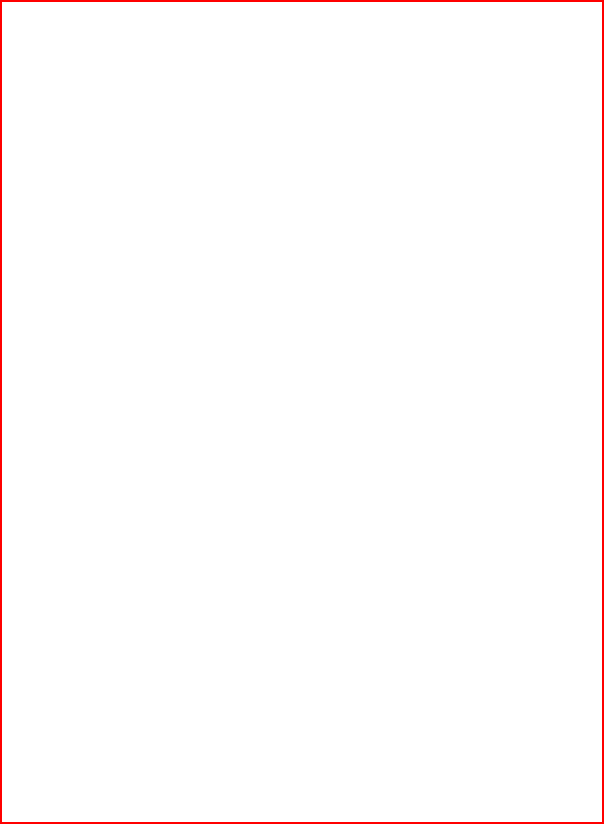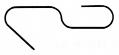Next > | MGA Vol. 1 | Highlights | Contents | 08 | 09 | 010 | 012 | 014 | 015 | 129 | 130 | 131 | 132 | < Back010-012 xxx

Math Games & Activities, Vol. 1

Balance Beam Cutout (p. 129)

The beam for a math balance.

To make a math balance, suspend the beam from a large paper cup as shown in Figures 3 and 4. (An alternative to the cup is an empty milk carton.)Figure 5

The major use of a math balance is to develop the concept of equality by having elementary school students use one to solve problems like the ones on pages 130, 131, and 132. Since a math balance is self-correcting, and the problems are non-verbal, even very young children can solve them and, in doing so, not only experience equality in a meaningful way, but are introduced to the basic facts for addition and multiplication.

A math balance can also be used to teach problem solving by having children solve teacher-directed problems like the following:Figure 3. Front

Figure 4. Side

If the beam is off balance to begin with, trim the heavy end of it with scissors. For weights, use partially straightened paper clips as shown in Figure 5.